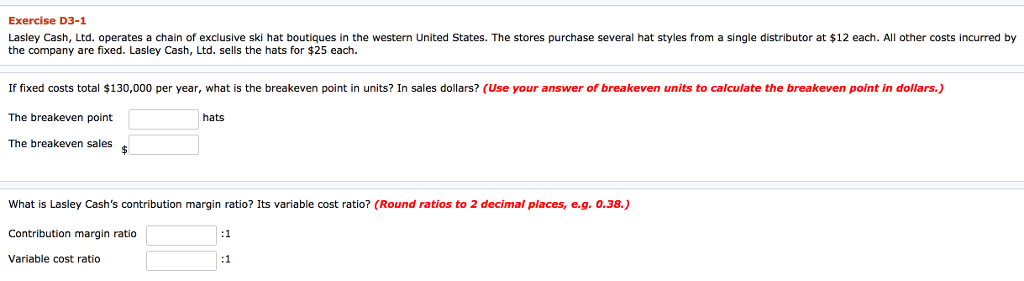# Question & Answer: nited States. The stores purchase several hat styles from a single distributor at \$12 each. All other costs in…..Lasley Cash, Ltd. operates a chain of exclusive ski hat boutiques in the western United States. The stores purchase several hat styles from a single distributor at \$12 each. All other costs incurred by the company are fixed. Lasley Cash, Ltd. sells the hats for \$25 each. If fixed costs total \$130,000 per year, what is the breakeven point in units? In sales dollars? (Use your answer of breakeven units to calculate the breakeven point in dollars.) The breakeven point hats The breakeven sales \$ What is Lasley Cash’s contribution margin ratio? Its variable cost ratio? (Round ratios to 2 decimal places, e.g. 0.38.) Contribution margin ratio: 1 Variable cost ratio: 1

Don't use plagiarized sources. Get Your Custom Essay on
Question & Answer: nited States. The stores purchase several hat styles from a single distributor at \$12 each. All other costs in…..
GET AN ESSAY WRITTEN FOR YOU FROM AS LOW AS \$13/PAGE

Contribution Margin Per Unit = Selling Price Per Unit – Variable Cost  Per Unit

= \$ 25 – \$ 12

= \$ 13

Break Even Point = Fixed cost / Contribution Margin Per Unit

= \$ 130,000 / \$ 13

= 10,000 Hats

The Break Even Sales = Break Even Point in units * Selling Price Per Unit

= 10,000 Hats * \$ 25

= \$ 250,000

Hence the correct answer is :

 The Break Even Point 10,000 Hats The breakeven Sales \$ 250,000

Contribution Margin Ratio =Contribution Margin Per Unit / Selling Price Per Unit

= \$ 13/ \$ 25

0.52 : 1

Variable Cost Ratio = Variable Cost Per Unit / Selling Price Per Unit

= \$ 12/ \$ 25

0.48 : 1